Function Repository Resource:

# InfiniteCatacausticCurve

Compute the catacaustic of a curve at infinity

Contributed by: Jan Mangaldan
 ResourceFunction["InfiniteCatacausticCurve"][c,θ,t] gives an equation for the catacaustic at infinity of a curve c specified parametrically with respect to a direction angle θ in terms of the variable t.

## Details and Options

A catacaustic at infinity is a curve that is the envelope of rays emanating from a point at infinite distance producing parallel rays for a given mirror shape.

## Examples

### Basic Examples (2)

The catacaustic at infinity for a parabola, with rays perpendicular to the parabola's axis:

 In:=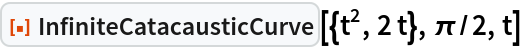Out=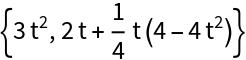Plot the parabola and the catacaustic (Tschirnhausen cubic) together:

 In:=Out=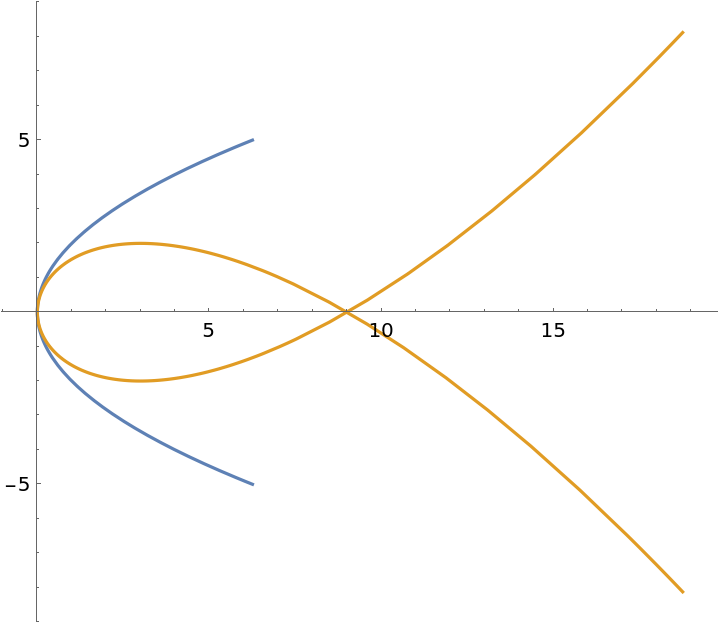The catacaustic at infinity for a circle:

 In:=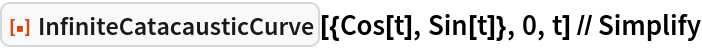Out=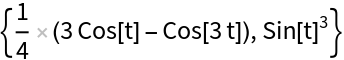Plot the circle and the catacaustic (nephroid) together:

 In:=Out=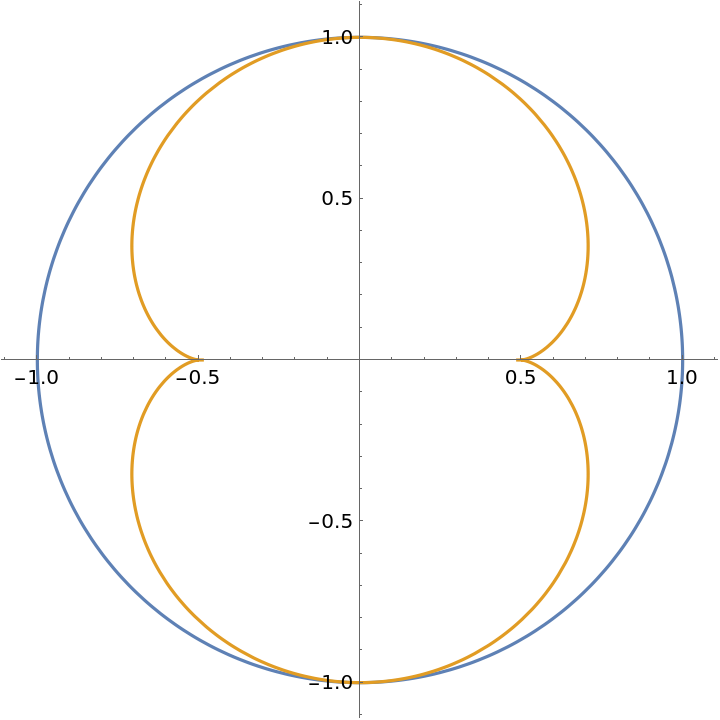### Neat Examples (2)

Parametric equations for a deltoid:

 In:=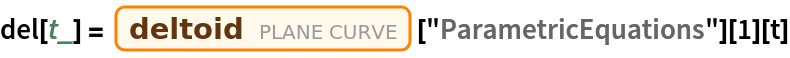Out=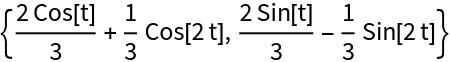Demonstrate the effect of changing θ in InfiniteCatacausticCurve:

 In:=Out=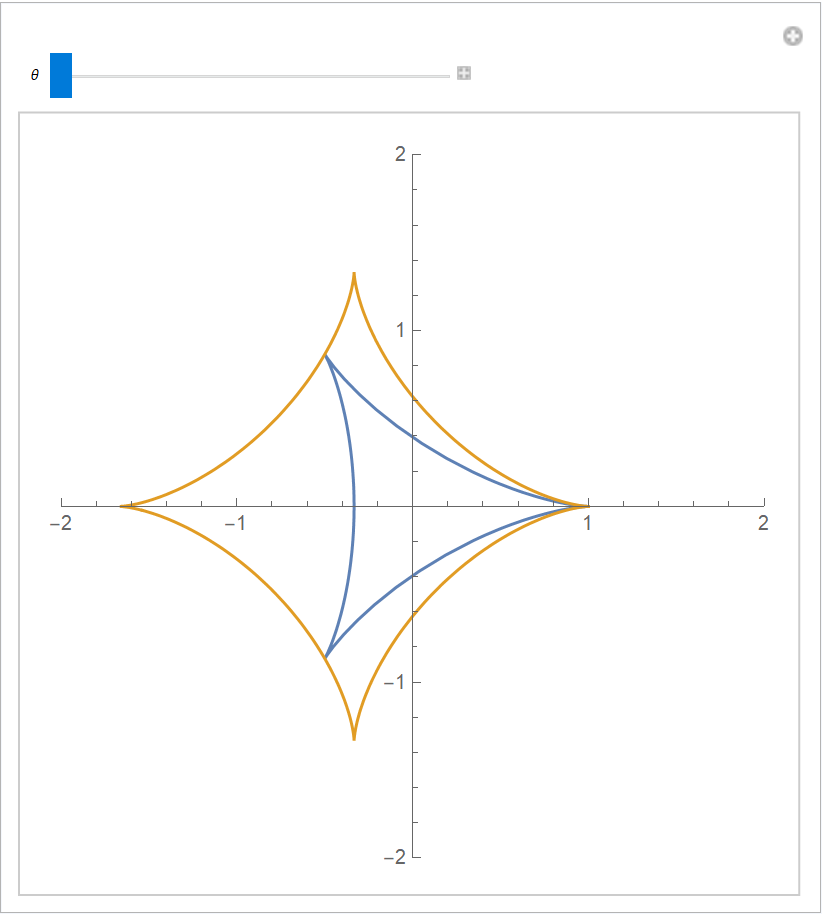## Version History

• 1.0.0 – 15 February 2021